upm  1.7.1 Sensor/Actuator repository for libmraa (v2.0.0)
ECS1030 Class Reference

API for the ECS1030 Non-Invasive Current/Electricity Sensor. More...

## Detailed Description

This non-invasive current sensor can be clamped around the supply line of an electrical load to tell you how much current is passing through it. It does this by acting as an inductor and responding to the magnetic field around a current-carrying conductor. This particular current sensor measures a load up to 30 A, which makes it great for building your own energy monitors.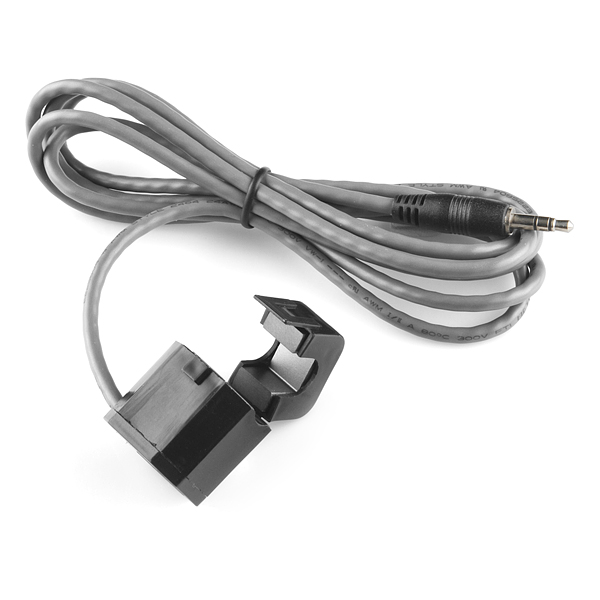ECS1030 Sensor image provided by SparkFun* under CC BY 2.0.

int
main(int argc, char** argv)
{
upm::ECS1030 sensor(0);
signal(SIGINT, sig_handler);
while (!is_running) {
std::cout << "I = " << sensor.getCurrency_A() << ", Power = " << sensor.getPower_A()
<< std::endl;
std::cout << "I = " << sensor.getCurrency_B() << ", Power = " << sensor.getPower_B()
<< std::endl;
}
std::cout << "exiting application" << std::endl;
return 0;
}

## Public Member Functions

ECS1030 (int pinNumber)

~ECS1030 ()

double getCurrency_A ()

double getPower_A ()

double getCurrency_B ()

double getPower_B ()

std::string name ()

## Static Public Attributes

static const uint8_t DELAY_MS = 20000 / NUMBER_OF_SAMPLES

static const uint8_t VOLT_M = 5.1 / 1023

static const uint8_t R_LOAD = 2000.0 / CURRENT_RATIO

## Constructor & Destructor Documentation

 ECS1030 ( int pinNumber )

Instantiates an ECS1030 object

Parameters
 pinNumber Number of the data pin
 ~ECS1030 ( )

ECS1030 object destructor; basically, it closes the GPIO.

## Member Function Documentation

 double getCurrency_A ( )

Returns electric current data for a sampled period

Here is the caller graph for this function: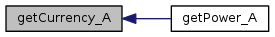double getPower_A ( )

Returns power data for a sampled period

Here is the call graph for this function: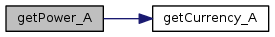double getCurrency_B ( )

Returns electric current data for a sampled period

Here is the caller graph for this function: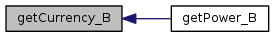double getPower_B ( )

Returns power data for a sampled period

Here is the call graph for this function: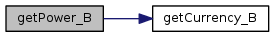std::string name ( )
inline

Returns the name of the component

The documentation for this class was generated from the following files: